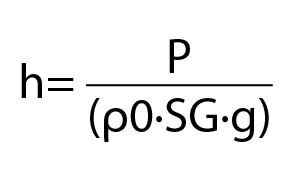# Pressure to Liquid Level Calculator

This calculator can be used to calculate the level of a liquid in a tank when the hydrostatic pressure in the tank and the specific gravity of a liquid are known.

The output of this calculator is the height difference between the top of the liquid and the location of the pressure measurement. This calculation assumes that the top of the fluid column is vented to atmosphere. If the vessel is not vented, then it is necessary to account for the actual pressure above the liquid.

The formula used is:Where,

h is the height of the liquid (m)

P is the pressure in the vessel (Pa)

ρ0 is the density of water (1000kg m^(-3) )

SG is the specific gravity of the liquid being measured

g is the acceleration due to gravity (typically 9.80665m s^(-2))

This calculator is provided for reference only. We have made every effort to ensure the accuracy of the information presented above, however Strain Measurement Devices assumes no liability for damages resulting from the use of this resource. Please use at your own risk and verify critical calculations independently.